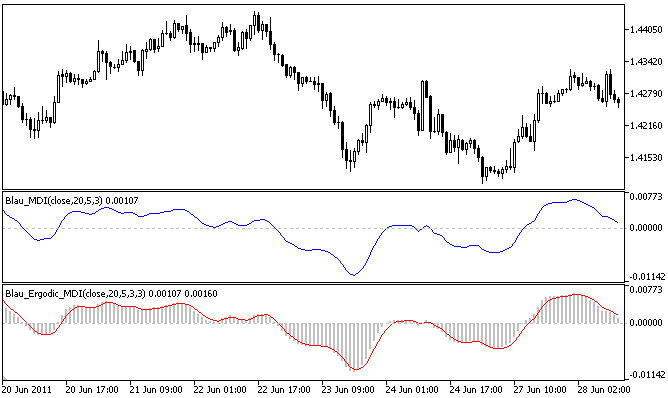Interesting script?
So post a link to it -
let others appraise it

You liked the script? Try it in the MetaTrader 5 terminal# Ergodic Mean Deviation Index Oscillator Ergodic_MDI - indicator for MetaTrader 5

Views:
3322
Rating:
Published:
2011.06.28 19:21
Updated:
2016.11.22 07:32

Author: Andrey N. Bolkonsky

Ergodic MDI-Oscillator by William  Blau is based on Mean Deviation Index (see Momentum, Direction, and Divergence: Applying the Latest Momentum Indicators for Technical Analysis).

Usage:
• WilliamBlau.mqh must be placed in terminal_data_folder\MQL5\Include\
• Blau_Ergodic_MDI.mq5 must be placed in terminal_data_folder\MQL5\Indicators\Ergodic MDI-Indicator by William Blau

Calculation:

Ergodic Mean Deviation Oscillator is calculated as follows:

Ergodic_MDI(price,r,s,u) = MDI(price,r,s,u)
SignalLine(price,r,s,u,ul) = EMA( Ergodic_MDI(price,r,s,u) ,ul)

where:

• Ergodic_MDI() - Ergodic (mean deviation indicator MDI(price,r,s,u));
• SignalLine() - Signal Line - exponentially smoothed moving average of a period ul, applied to ergodic;
• ul - period of EMA of a Signal Line.
Input paramters:
• graphic plot #0 - Ergodic (Mean Deviation Indicator):
• r - period of the 1st EMA, applied to price (by default r=20);
• s - period of the 2nd EMA, applied to mean deviation (by default s=5);
• u - period of the 3rd EMA, applied to result of the 2nd smoothing (by default u=3);
• graphic plot #1 - Signal Line:
• ul - EMA period of a Signal Line, applied to ergodic (by default ul=3);
• AppliedPrice - price type (by default AppliedPrice=PRICE_CLOSE).
Note:
• r>1;
• s>0, u>0. If r, s or u =1, smoothing is not used;
• ul>0. If ul=1, the Signal Line and Mean Deviation Index are the same;
• Min. rates=(r+s+u+ul-4+1).

Translated from Russian by MetaQuotes Software Corp.
Original code: https://www.mql5.com/ru/code/374Mean Deviation Index Blau_MDI

Mean Deviation Index (MDI) by William Blau.Stochastic Momentum Oscillator Blau_SM_Stochastic

Stochastic Momentum Oscillator by William Blau.Moving Averages Convergence/Divergence Indicator Blau_MACD

Moving Averages Convergence/Divergence Indicator by William Blau.Ergodic MACD Oscillator Blau_Ergodic_MACD

Ergodic MACD Oscillator by William Blau.